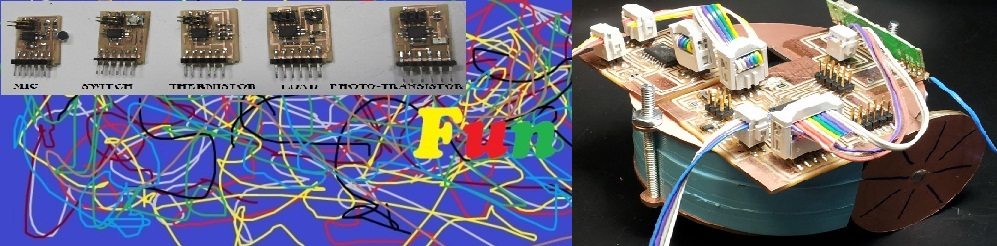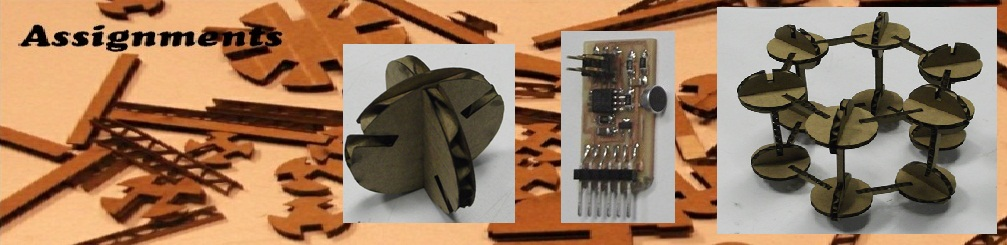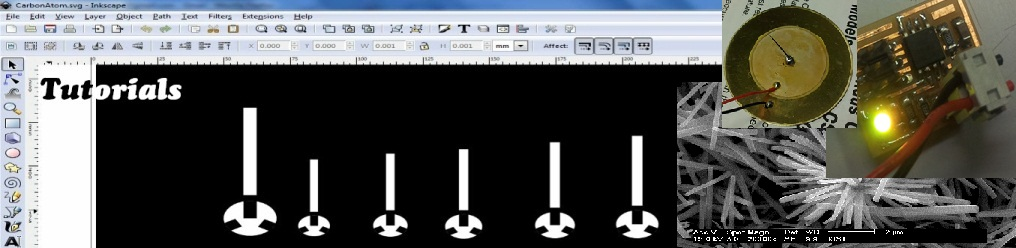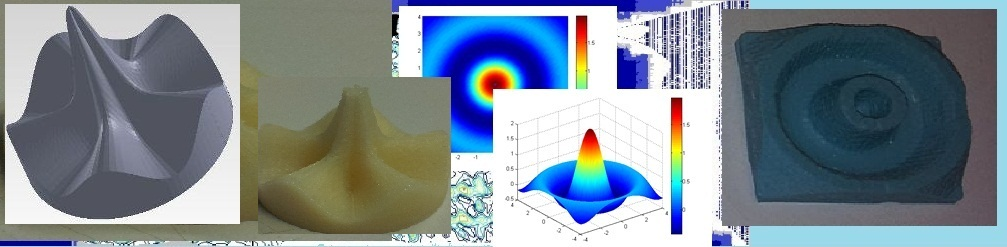# Assignments

## Molding & Casting

This weeks assignment was molding and casting. Mold was prepaird by milling wax and the cast was prepaired using hydro-stone.

## Idea

The aim of this assignment was to build curve that is represented by a single mathematical function. Unlike the CAD method, this method is more programmable, where one can design any function in fom a mathematical equation.

## Design

The design process involved imagination (most powerfull graphic card) of the curve and then implimanting it in mathematical equation as shown below.

THINK -> MATH (cos(5\$) + 6exp(-r^2)) ->

PLOT->GrayScale -> Modela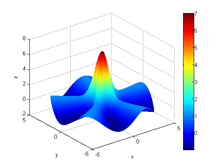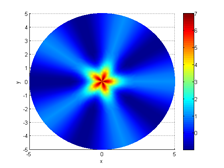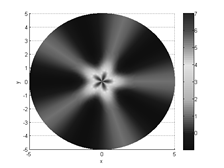## First Attempt

Since the mold was deep, the sholder of the tool collided with the edge of the mold. Which stopped the modela leaving the mold half done.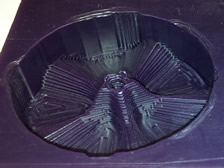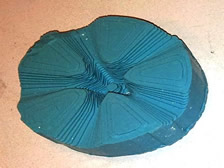I could not surface finish the mold because, the surface finish mode assume there is no material but since the mold was only half finished so there was some part of the mold which was not milled.

I made a mistake also, If you see the design (2D grapy plot) the center is black which will be milled by the tool and since the tool has finite width less then the feature size in the design (mathematics not follow the design rule) I end up with the tapered peak.

Mathematics has singularity but real word doesn't

## Second Attempt

To avoid problems mentioned above I decided to change the curve and compliment the final gray scale image to get maximum at the center.

Equation.

R = sqrt(X.*X + Y.*Y);
Z = sin(2*R)./(R + 0.001)
;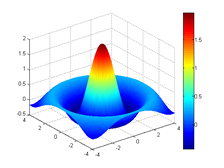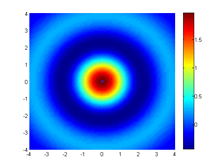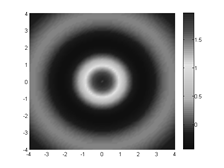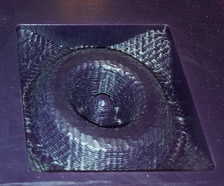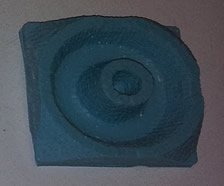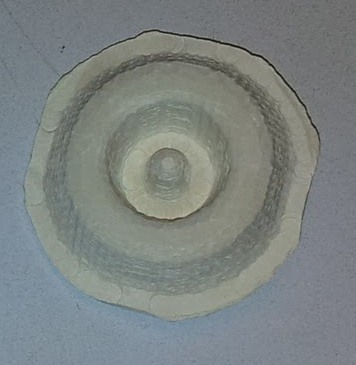You can see that the mold is exactly not as it should be (colored graph). The reason is that when I transfered from Color Top View image (3D matrix) to grayscale (2D matrix), I lost some information in form of each pixel height. The lession I learnt from this is that one should not convert color image to grayscale, instead should yous height map to gray scale image.

Casting

For casting, I used drystone and added ZnO nanoparticles (phosphor nanomaterial) in it.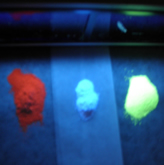So that when I expose my cast in UV light it will phosphour with different colors. But since the quatity I added was very small only red color appeared when exposed to UV and that too was not much detectable with camera.

.

.